Which of the following relations is correct for the root-mean-square speed of gas at temperature T?

Where, k= Boltzmann constant, and m= mass of a molecule.

(A)     (B)

(C)     (D)

Concept Questions :-

Types of velocity
High Yielding Test Series + Question Bank - NEET 2020

Difficulty Level:

A thermally insulated piston divides a container into two compartments.  Volume, temperature, and pressure in the right compartment are 2V, T, and 2P, while in the left compartment the respective values are V, T, and P.  If the piston can slide freely, then in the final equilibrium position, the volume of the right-hand compartment will be-

(1) V            (2) $\frac{3V}{5}$          (3) $\frac{9V}{4}$          (4) $\frac{12V}{5}$

Concept Questions :-

Ideal gas
High Yielding Test Series + Question Bank - NEET 2020

Difficulty Level:

The graph which represents the variation of mean kinetic energy of molecules with temperature $t°C$ is ?

(1)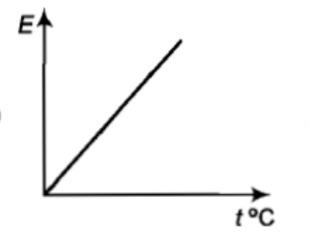(2)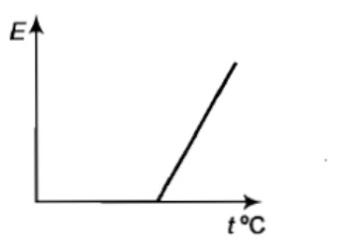(3)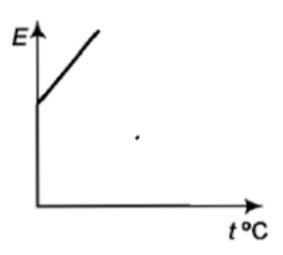(4)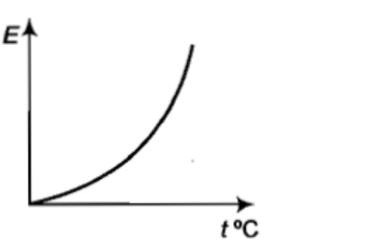Concept Questions :-

Kinetic energy of gas
High Yielding Test Series + Question Bank - NEET 2020

Difficulty Level:

${V}_{rms},{V}_{av},{V}_{mp}$ are root mean square, average and most probable speeds of molecules of a gas obeying Maxwellian velocity distribution. Which of the following statements is correct?

1. ${V}_{rms}<{V}_{av}<{V}_{mp}$

2. ${V}_{rms}>{V}_{av}>{V}_{mp}$

3.

4. ${V}_{avg}>{V}_{rms}<{V}_{mp}$

Concept Questions :-

Types of velocity
High Yielding Test Series + Question Bank - NEET 2020

Difficulty Level:

Gas is found to obey the law ${P}^{2}V$ = constant. The initial temperature and volume are ${T}_{0}$ and ${V}_{0}$. If the gas expands to a volume $3{V}_{0}$, its final temperature becomes:

(a) $\frac{{T}_{0}}{3}$           (b) $\frac{{T}_{0}}{\sqrt{3}}$

(c) $3{T}_{0}$           (d) None of these

Concept Questions :-

Ideal gas
High Yielding Test Series + Question Bank - NEET 2020

Difficulty Level:

For a gas, the r.m.s speed at 800 K is:

(1) Four times the value at 200 K

(2) Half the value at 200 K

(3) Twice the value at 200 K

(4) Same as at 200 K

Concept Questions :-

Types of velocity
High Yielding Test Series + Question Bank - NEET 2020

Difficulty Level:

The average kinetic energy of a helium atom at $30°C$ is:                                                                                           [MP PMT 2004]

(1) Less than 1 eV

(2) A few KeV

(3) 50-60 eV

(4) 13.6 eV

Concept Questions :-

Kinetic energy of gas
High Yielding Test Series + Question Bank - NEET 2020

Difficulty Level:

The mean translational kinetic energy of a perfect gas molecule at the temperature T Kelvin is:

(1) $\frac{1}{2}kT$         (2) kT             (3) $\frac{3}{2}kT$            (4) 2 kT

Concept Questions :-

Kinetic energy of gas
High Yielding Test Series + Question Bank - NEET 2020

Difficulty Level:

Choose the correct option related to the specific heat at constant volume ${C}_{V}$ -

(1) ${C}_{V}=\frac{1}{2}fR$              (2) ${C}_{V}=\frac{R}{\gamma -1}$

(3) ${C}_{V}=\frac{{C}_{P}}{\gamma }$                (4) All of these

Concept Questions :-

Specific heat
High Yielding Test Series + Question Bank - NEET 2020

Difficulty Level:

The curve between absolute temperature and ${{v}_{rms}}^{2}$ is:

(a)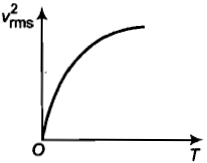(b)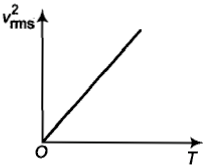(c)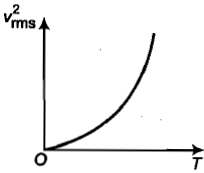(d)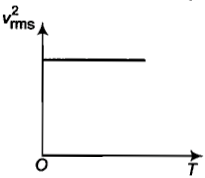Concept Questions :-

Types of velocity